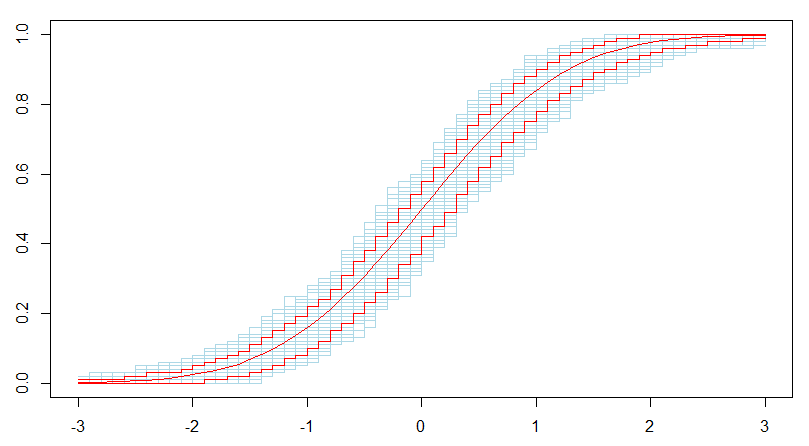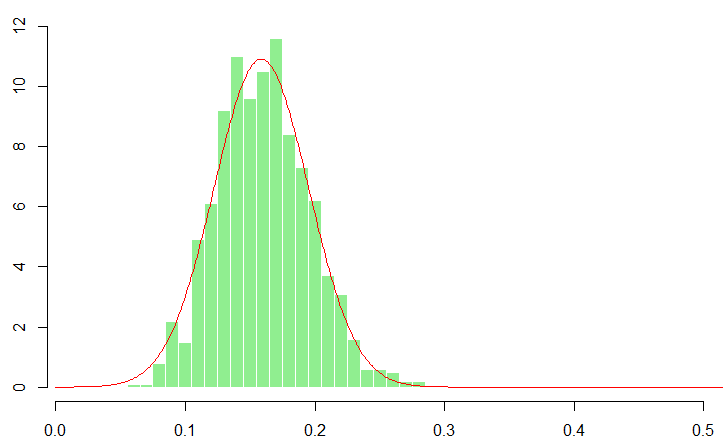Want to share your content on R-bloggers? click here if you have a blog, or here if you don't.

Last week, in our mathematical statistics course, we’ve seen the law of large numbers (that was proven in the probability course), claiming that

given a collection  of i.i.d. random variables, with

To visualize that convergence, we can use

```> m=100
> mean_samples=function(n=10){
+   X=matrix(rnorm(n*m),nrow=m,ncol=n)
+   return(apply(X,1,mean))
+ }
> B=matrix(NA,100,20)
> for(i in 1:20){
+   B[,i]=mean_samples(i*10)
+ }
> colnames(B)=as.character(seq(10,200,by=10))
> boxplot(B)```

It is possible to visualize also the  bounds (used in the central limit theorem to get a limiting non degenerated distribution)

```> u=seq(0,21,by=.2)
> v=sqrt(u*10)
> lines(u,1.96/v,col="red")
> lines(u,-1.96/v,col="red")```Yesterday, we’ve been discussing properties of the empirical cumulative distribution function,

We’ve seen Glivenko-Cantelli theorem, which states that (under mild assumptions)

To visualize that convergence use the following code. Here I use the trick

to get the maximum (componentwise) between two matrices

```> m=100
> inf_sample=function(n=10){
+ X=matrix(rnorm(n*m),nrow=m,ncol=n)
+ Xs=t(apply(X,1,sort))
+ Pe_inf=matrix(rep((0:(n-1))/n,
+ each=m),nrow=m,ncol=n)
+ Pe_sup=matrix(rep((0:n)/n,each=m),
+ nrow=m,ncol=n)
+ Pt=pnorm(Xs)
+ D1=abs(Pe_inf-Pt)
+ D2=abs(Pe_sup-Pt)
+ Df=(D1+D2)/2+abs(D2-D1)/2
+ return(apply(Df,1,max))
+ }
> B=matrix(NA,100,20)
> for(i in 1:20){
+   B[,i]=inf_sample(i*10)
+ }
> colnames(B)=as.character(seq(10,200,by=10))
> boxplot(B)```We have also discussed the pointwise asymptotic normality of the empirical cumulative distribution function

Here again, it is possible to visualize it. The first step is to compute several trajectories for empirical cumulative distribution function

```> u=seq(-3,3,by=.1)
> plot(u,u,ylim=c(0,1),col="white")
> M=matrix(NA,length(u),1000)
> for(m in 1:1000){
+ n=100
+ x=rnorm(n)
+ Femp=Vectorize(function(t) mean(x<=t))
+ v=Femp(u)
+ M[,m]=v
+ lines(u,v,col='light blue',type="s")
+ }```

Note that we can compute (pointwise) confidence bands

```> lines(u,apply(M,1,mean),col="red",type="l")
> lines(u,apply(M,1,function(x) quantile(x,.05)),
+ col="red",type="s")
> lines(u,apply(M,1,function(x) quantile(x,.95)),
+ col="red",type="s")```Now, if we focus on one specific point, we can visualize the asmptotic normality (i.e. the almost normality when we have a sample of size 100)

```> x0=-1
> y=M[which(u==x0),]
> hist(y,probability=TRUE,
+ breaks=seq(.015,0.55,by=.01))
> vu=seq(0,1,by=.001)
> lines(vu,dnorm(vu,pnorm(x0),
+ sqrt((pnorm(x0)*(1-pnorm(x0)))/100)),
+ col="red")```